### Integer Points

Source: Mailed to me by Sudeep Kamath (PhD Student, UC at Berkeley, EE IITB Alumnus 2008) - He found it at http://puzzletweeter.com/

Problem:

An integer point in a plane is a point whose coordinates are integers.

Suppose we arbitrarily choose 5 integer points in a plane.

Show that we can always find 2 among these 5 integer points such that the line segment joining the 2 points contains at least 1 more integer point.

1.Consider the case of all 5 points on a circle.
The argument does not hold.

1.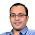@Shashank Kumar
Sorry. How does point being on a circle make any difference? We are talking about 5C2 line segments.

2.He's right; there's no line that would contain 3 of such points.
I was going with a quadratic function., say for instance points

(2;4), (3, 9), (4, 16), (5, 25)

No line crossing two of this points will cross a third one.

3.I think you misunderstood the problem @Shashank Kumar; the segment will contain an integer point, not necessarily one of those 5 you've chosen.

4.@Shashank Kumar, how did you put 5 "integer points" on a circle?

5.@Shashank I believe you've read the question incorrectly. It does not suggest that one of the other five integer points must be on the line segment, but rather there is some integer point out of all possible integer points on at least one of the line segments between each two points.

2."1 more integer point" suggests the point needs to be different from all the 5 original points, which does not need to be the case.

3.I just randomly drew on my notepad. Counterexample: (0, 2), (1, 2), (1, 3), (2, 0), (3, 1)

1.the line from (0,2) and (2,0) passes through (1,1)

2.(0,2) and (2,0) intersect at (1,1)

3.(0,2) and (2,0) intersect at (1,1)

(1,3) and (3,1) intersect at (2,2)

4.Label the points xi, yi. Consider { ( xi mod 2, yi mod 2 ) }. These can fall in four different buckets, so there is a pair (x0, y0), (x1, y1) that fall in the same bucket. Hence x1-x0 = 0 and y1-y0 = 0 mod 2. This implies x1-x0=2k and y1-y0=2j for some integers k, j (at least one of k, j > 0; otherwise, the two points are equal). So x0+k, y0+j is an integer point on the line segment (x0,y0) to (x1,y1).

5.Can you choose 5 integer points on a circle ?

6.There are 4 parities - {Odd, Odd}, {Even, Odd}, {E, E}, {O, E}.

By Pigeon Hole Principle, atleast 2 points must have the same parity.
The mid-point has to be an integer point.

1.awesome.......

2.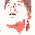indeed! so simple and elegant.

7.I don't think it's correct. Consider the case of a 5-points star. Pick a point (anyone will do). Draw four lines joining the others points; no line include three points, and that are all the lines you can draw.

1.Can you find 5 integer points that can form a star without having a line go either vertical or horizontal (therefore necessarily including other integer points)? I don't think it's possible.

8.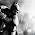Considering the parity of the integer coordinates, there are 4 possibilities - (odd, odd), (odd, even), (even, odd), (even, even). By pigeonhole principle, amongst the 5 randomly chosen points, there will exist at least two points with same parity say (x1, x2) and (y1, y2). Then ((x1+y1)/2, (x2+y2)/2) is also an integer point.

1.I guess this is the solution in short.
@Balaji: the integer point falling on the line connecting (x1,y1) and (x2,y2) does not have to be one of the five chosen points. This is my understanding of the puzzle.

9.@Arun Iyer. But is it necessary that my fifth point my be the mid point?

1.The mid point is an integer co-ordinate. There may be more, but we just need to show existence of one.

10.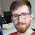Might want to add the word "distinct" to your problem: "Suppose we arbitrarily choose 5 *distinct* integer points in a plane." Otherwise there is an edge case which fails, where all five points are the same. Eg A = (0,0), B = (0,0), C = (0,0), D = (0,0), E = (0,0) Thus no line can be created which intersects an integer point that isn't included on the list.

11.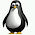make sense, 4 integer point is the largest number (on 2D plane) such that there is no 3rd point coming in between (assuming all the 4 integer point) are exactly next to each other (+1 distance away). If u add in one more point, it has to intersect one of the existing point.

12.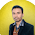13.Out of the 5 points at least 3 of them will be having odd x-co-ordinates or even x-co-ordinates, chose those three. Out of the chosen 3 points at least 2 will have odd y-co-ordinates or even co-ordinates, chose those two points and their x-co-ordinate and y-co-ordinates are of same parity, so the midpoint of the line joining is an integer point as well.

14.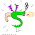https://dl.dropboxusercontent.com/u/23136754/BS.png

1.15.wlog, let one of the points be the origin (0,0)
The remaining 4 points will be of the form (E,E), (E,O), (O,E) or (O,O) where E denotes a +ve or -ve even number, O denotes a +ve or -ve odd number.
If we have a point of the form (E,E), the mid point of the origin and the point will be (E/2,E/2) - an integer point.
If we do not have (E,E) among the remaining 4 points, atleast 1 of of the other 3 types of points will have to be repeated (Pigeon Hole). The mid-point between 2 points of the same type will be an integer point (eg: mid-point of 2 (E,O)s is ((E1+E2)/2,(O1+O2)/2), which is an integer point).
Hence proved :)

16.You have 5 integral positions. Let them be (x_1, y_1), (x_2, y_2), (x_3, y_3), (x_4, y_4), (x_5, y_5). Now you consider only the y coordinate. By Pigeonhole principle, out of these 5 y's three will have the same parity. Now for those three look at their x coordinates. Again, by pigeonhole principle, out of these 3 x's two will have the same parity. Now these two integral positions have same parity of their respective coordinates. Let them be (x_p,y_p) and (x_q,y_q).
Now taking the midpoint:
((x_p + x_q)/2, (y_p + y_q)/2)
This position will be integral as both the above quantities are divisible by 2.

17.X coordinate can be odd or even
Y coordinate can be odd or even

(X,Y) has 4 options -
1. o,o
2. o,e
3. e,o
4. e,e

But we have 5 points. So atleast 2 are of the same type. Their midpoint is integer :)